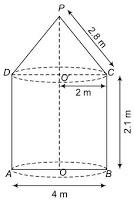Newbie

# A tent is in the shape of a cylinder surmounted by a conical top. If the height and diameter of the cylindrical part are 2.1 m and 4 m respectively, and the slant height of the top is 2.8 m, find the area of the canvas used for making the tent. Also, find the cost of the canvas of the tent at the rate of Rs 500 per m2. (Note that the base of the tent will not be covered with canvas.)Q.7

• 0

What is the best method of ncert class 10 surface areas and volumes chapter of exercise 13.1 question number 7 , Also give me the best and easiest solution of this question. Find the best method of this question.A tent is in the shape of a cylinder surmounted by a conical top. If the height and diameter of the cylindrical part are 2.1 m and 4 m respectively, and the slant height of the top is 2.8 m, find the area of the canvas used for making the tent. Also, find the cost of the canvas of the tent at the rate of Rs 500 per m2. (Note that the base of the tent will not be covered with canvas.)

Share

1. It is known that a tent is a combination of cylinder and a cone.From the question we know that

Diameter = 4 m

Slant height of the cone (l) = 2.8 m

Radius of the cone (r) = Radius of cylinder = 4/2 = 2 m

Height of the cylinder (h) = 2.1 m

So, the required surface area of tent = surface area of cone + surface area of cylinder

= πrl+2πrh

= πr(l+2h)

= (22/7)×2(2.8+2×2.1)

= (44/7)(2.8+4.2)

= (44/7)×7 = 44 m2

∴ The cost of the canvas of the tent at the rate of ₹500 per mwill be

= Surface area × cost per m2

44×500 = ₹22000

So, Rs. 22000 will be the total cost of the canvas.

• 0